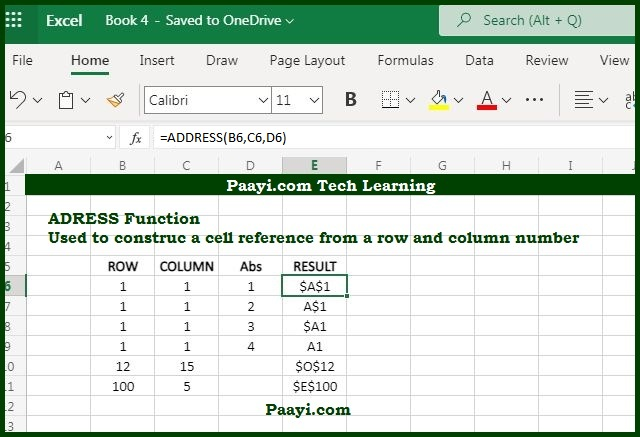# Learn How to use the Microsoft Excel ADDRESS function

Written by | 0 Comments | 741 Views

In this article, you will learn how to use the Microsoft excel address function. First, understand what is Microsoft excel address function is and then understand how you can implement it in your Microsoft excel.

The Microsoft Excel ADDRESS function's main objective is to create a cell address from a row and column provided. That means with the help of the ADDRESS function, you can return the value as an address of a cell according to the given row and column number. You can able to return the address in the relative or absolute format and also able to construct the cell reference in the formula.

The return value will be the cell address in the current or given worksheet.

= ADDRESS(row-num, col-num, [abs-num], [a1], [sheet])

Where the arguments:

• row-num: It is the row number that you can use in the cell address.
• col-num: It is the column number that you can use in the cell address.
• abs-num: This is the address type, relative or absolute; the default is absolute (optional).
• a1: The reference style (Optional).
• sheet: The name of the worksheet which you want to use defaults to the current sheet.

### How to Use Microsoft Excel ADDRESS Function?As we know, the ADDRESS function is used to create an address from a given row or column number; let's see few examples below:

• = ADDRESS(1, 1) - This will return the value \$A\$1
• = ADDRESS(1, 1, 5) - This will return the value A1.